Problem 1:

What is?

Solution:

Use a change of variables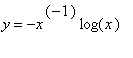to make the cosine linear in the variable of integration.

 > restart;

 > ti:= time(): Digits:= 20:

 > J:= Int(x^(-1)*cos(x^(-1)*ln(x)),x=epsilon..1);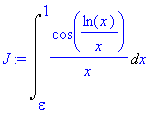> alias(W=LambertW): xs:= solve(y=-x^(-1)*ln(x),x);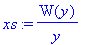> simplify(PDEtools[dchange](x=xs,J));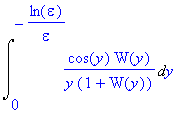Of course,.

 > integrand:= cos(y)*LambertW(y)/(1+LambertW(y))/y;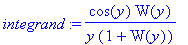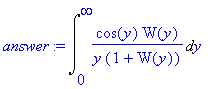This is still not a very pleasant oscillatory integral, although the singularity at 0 is removable.  We break it up into 3 pieces.  For y from 0 to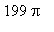, use ordinary numerical integration, (but on the original form of the integral, to avoid the apparent singularity at y=0 as well as not having to evaluate W numerically; and using the _Dexp method, as otherwise Maple will have trouble because it's close to the singularity at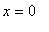).

 > Int(1/x * cos(ln(x)/x) , x=subs( y=199*Pi, xs ) ... 1 ); r1:= evalf(Int(op(%),_Dexp));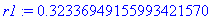For each interval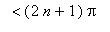for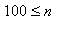,  write the integral as.  Expand this in an asymptotic series in n and integrate term-by-term.   The terms for even n vanish by symmetry.

 > map(factor,asympt(cos(t)*W(t+2*n*Pi)/(t+2*n*Pi)/(1+W(t+2*n*Pi)), n, 8));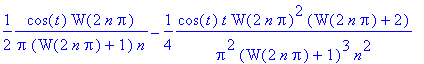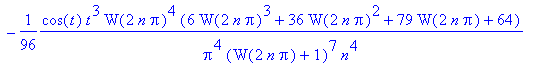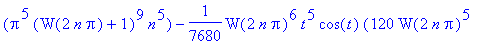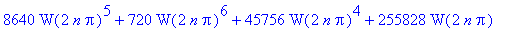> J2:= map(factor,map(int, eval(%,O=0), t=-Pi..Pi));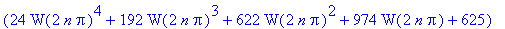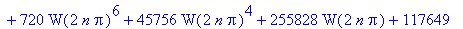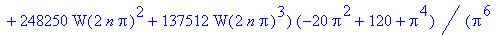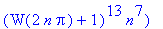> L:= convert(%,list): evalf(eval(L,n=100));Apparently by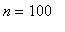we only need the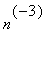andterms.  We'll use these for n from 100 to 1000.For n > 1000, use the asymptotics of the Euler-Maclaurin form for the summation of theandterms.

 > J4:= map(factor,asympt(eulermac(J3,n),n));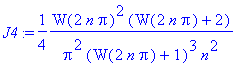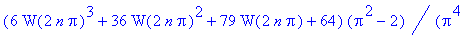> r3:= -evalf(eval(J4,{O=0, n=1001}));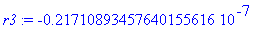> r:= r1+r2+r3;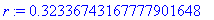Actual answer, as given by Trefethen to 40 digits:

0.3233674316 7777876139 9370087952 1704466510...

 > (time()-ti)*seconds;